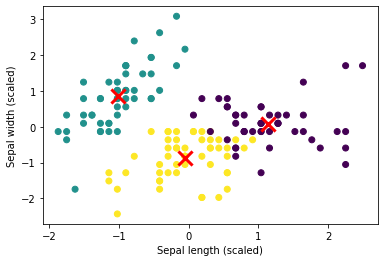In this post you can find how to build a simple machine learning model in Python. We will cover the basics of the ML model and how to build it quickly in a few lines of code.

Below you can find the full code of a machine learning model for classifying flowers based on their properties.

``````import seaborn as sns
from sklearn.model_selection import train_test_split
from sklearn.linear_model import LogisticRegression

X_train, X_test, y_train, y_test = train_test_split(iris.drop('species',axis=1), iris['species'], test_size=0.3, random_state=42)

model = LogisticRegression()
model.fit(X_train, y_train)

y_pred = model.predict(X_test)
``````

Below you can find an explanation of the code and details.

## Setup

We are using modules:

• `sklearn` - for the ML module
• `seaborn` - for the training and testing dataset

We import the required modules and methods. Next we read the iris.csv dataset:

sepal_length sepal_width petal_length petal_width species
0 5.1 3.5 1.4 0.2 setosa
1 4.9 3.0 1.4 0.2 setosa
2 4.7 3.2 1.3 0.2 setosa
3 4.6 3.1 1.5 0.2 setosa
4 5.0 3.6 1.4 0.2 setosa

The original dataset has 150 records.

## Dataset preparation

ML models needs data for training which has:

• input
• sepal_length
• sepal_width
• petal_length
• petal_width
• output
• species

To build training and testing dataset we will split original dataset in 2 parts by:

``````input_data = iris.drop('species',axis=1)
output = iris['species']
X_train, X_test, y_train, y_test = train_test_split(input_data, output, test_size=0.3, random_state=42)
``````

So we will have:

• X_train - 105 rows
• X_test - 45 rows
• y_train - 105 rows
• y_test - 45 rows

Example of X_train:

sepal_length sepal_width petal_length petal_width
81 5.5 2.4 3.7 1.0
133 6.3 2.8 5.1 1.5
137 6.4 3.1 5.5 1.8
75 6.6 3.0 4.4 1.4
109 7.2 3.6 6.1 2.5

and example of y_train:

``````81 	versicolor
133 	virginica
137 	virginica
75 	versicolor
109 	virginica
``````

So we feed the model the properties of each flower and what is the name of it. The initial classification or labeling was done manually.

## Training ML model

To train the model we will use `LogisticRegression()` and provide the training data:

``````model = LogisticRegression()
model.fit(X_train, y_train)
``````

## Testing model

Next we can test the machine learning model by:

``````y_pred = model.predict(X_test)
``````

We can check the results:

``````array(['versicolor', 'setosa', 'virginica', 'versicolor'...
``````

Now our machine learning model is able to classify flowers based on 4 inputs and return the species.

## Verifying test results

To verify the test results we will use Pandas to compare the values:

``````(df.iloc[y_test.index]['species'] == y_test.values).value_counts()
``````

which returns:

``````True	45
Name: species, dtype: int64
``````

So we have 100% percent accuracy in prediction of iris species.

## Visualizing data

We can use K-means to cluster data into groups and visualize it by:

• build the K-means model
• use 3 clusters
• visualize the results
``````from sklearn.datasets import load_iris
from sklearn.preprocessing import StandardScaler
from sklearn.cluster import KMeans
import matplotlib.pyplot as plt

X = iris.data

scaler = StandardScaler()
X_scaled = scaler.fit_transform(X)

kmeans = KMeans(n_clusters=3, random_state=42)
kmeans.fit(X_scaled)

plt.scatter(X_scaled[:, 0], X_scaled[:, 1], c=kmeans.labels_)
plt.scatter(kmeans.cluster_centers_[:, 0], kmeans.cluster_centers_[:, 1], marker='x', s=200, linewidths=3, color='r')
plt.xlabel('Sepal length (scaled)')
plt.ylabel('Sepal width (scaled)')
plt.show()
``````

The results is below: